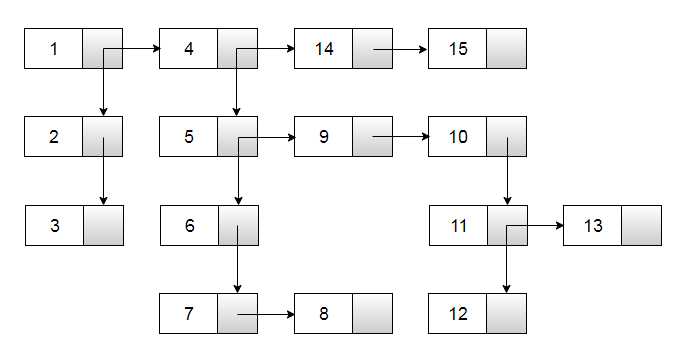# Flatten a multilevel linked list

Given a list which can grow in both horizontal and vertical directions (right and down), flatten it into a singly linked list. The conversion should be in such a way that down node is processed before the next node for any node.

This list is similar to the standard linked list except that it has one extra field down which points to a vertical list. The vertical list can have horizontal list attached to it and vice versa.

For example, consider below linked listThe Flattened list would be:

1 -> 2 -> 3 -> 4 -> 5 -> 6 -> 7 -> 8 -> 9 -> 10 -> 11 -> 12 -> 13 -> 14 -> 15 -> null

The idea is to use Recursion. We can recursively flatten a linked list by recursively flattening the down list first, followed by the next list. The flattened down list for a node is linked to the next pointer of that node, while the flattened next list for a node is linked to the next pointer of the last seen node.

This can be implemented as follows in C++:

Output:

The Original list is :
1 [ 2 [ 3 ]] 4 [ 5 [ 6 [ 7 8 ]] 9 10 [ 11 [ 12 ] 13 ]] 14 15

The Flattened list is :
1 -> 2 -> 3 -> 4 -> 5 -> 6 -> 7 -> 8 -> 9 -> 10 -> 11 -> 12 -> 13 -> 14 -> 15 -> null

The time complexity of above solution is O(n) and requires O(n) space for the call stack. We can further optimize the code by maintaining a tail pointer as we move along. This is demonstrated here.(1 votes, average: 5.00 out of 5)Loading...

Please use our online compiler to post code in comments. To contribute, get in touch with us.
Like us? Please spread the word and help us grow. Happy coding 🙂